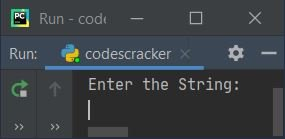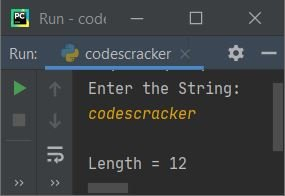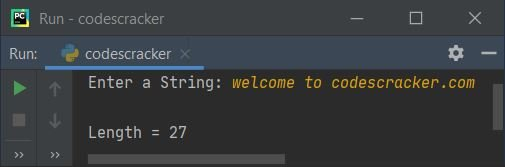# Python Program to Find Length of String

In this article, I've created some programs in Python, that find and prints length of string entered by user. Here are the list of approaches used:

• Find length of string using for loop
• Using while loop
• Using len() method
• Using user-defined function

## Find Length of String using for Loop

To find length of any string in Python, you have to ask from user to enter any string and then find its length in character-by-character manner, using for loop, like shown in the program given below:

```print("Enter the String: ")
text = input()

tot = 0
for ch in text:
tot = tot+1

print("\nLength =", tot)```

Here is its sample run:Now supply the input say codescracker as string and press `ENTER` key to find and print length of given string using for loop:When user enters a string say codescracker, then it gets stored in a variable named text. Now using for loop, the dry run of above program goes like:

• Initial value, tot=0
• Now the first character of text (that is, 'c') gets copied to ch variable, and program flow goes inside the loop, and the value of tot gets incremented by 1
• That is, tot+1 or 0+1 or 1 gets initialized to tot. So tot=1
• Now the second character of text (that is, 'o') gets copied to ch, and again program flow goes inside the loop and the value of tot again gets incremented by 1
• This process continues, until each and every character of text gets scanned
• In this way, after exiting from the loop, the variable tot holds the total number of characters available in the given string. That equals the length of string
• So print the value of tot on output, as length of string

## Find Length of String using while Loop

The question is, write a Python program to find length of a given string using while loop. Here is its answer:

```print("Enter a String: ", end="")
text = input()

tot = 0
while text[tot:]:
tot = tot+1

print("\nLength =", tot)```

Here is its sample run with string input welcome to codescracker.com:While slicing the string, the [tot:] refers to all characters from index number tot to last. For example if text = "codescracker" is a given string. Therefore at 0th index of text (text), 'c' is available, at 1st index of text (text), 'o' is available, and so on. So if tot=2, then text[2:] returns "descracker" (all characters from second index).

## Find Length of String using len()

This program is created using a predefined function named len(), that returns length of an object passed as its argument.

```print("Enter a String: ", end="")
text = input()

textlen = len(text)
print("\nLength =", textlen)```

## Find Length of String using Function

This is the final program on finding length of a given string in Python, created using a user-defined function named FindLenOfStr(). It returns the length of a value passed as its argument.

```def FindLenOfStr(x):
return len(x)

print("Enter a String: ", end="")
text = input()

print("\nLength =", FindLenOfStr(text))```

#### Same Program in Other Languages

Python Online Test

« Previous Program Next Program »